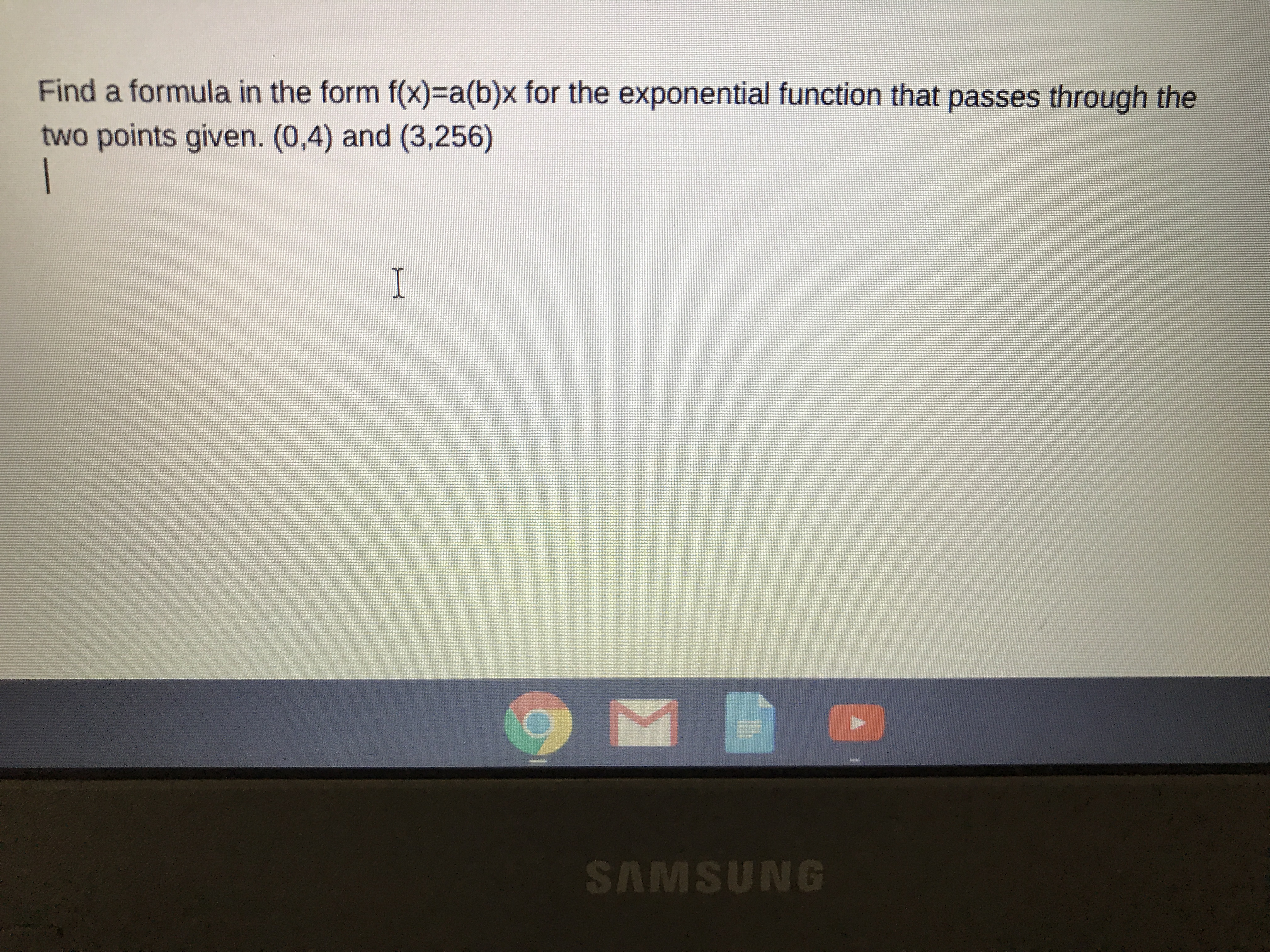# Find a formula in the form f(x)- a(b)x for the exponential function that passes through thetwo points given. (0,4) and (3,256)ISAMSUNG

Question
73 views

f(x)=a(b)x

the x at the end of that formula is an exponent of b , i believehelp_outlineImage TranscriptioncloseFind a formula in the form f(x)- a(b)x for the exponential function that passes through the two points given. (0,4) and (3,256) I SAMSUNG fullscreen
check_circle

Substitute given two points to solv...

### Want to see the full answer?

See Solution

#### Want to see this answer and more?

Solutions are written by subject experts who are available 24/7. Questions are typically answered within 1 hour.*

See Solution
*Response times may vary by subject and question.
Tagged in

### Functions